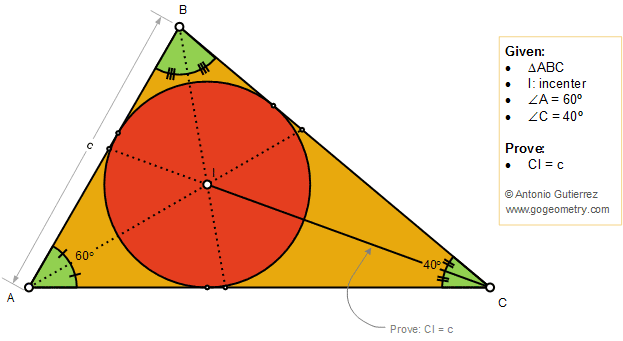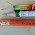Tuesday, September 25, 2018

Geometry Problem 1388: Triangle 40-60-80 degree, Incenter, Congruence

Geometry Problem. Post your solution in the comment box below.
Level: Mathematics Education, High School, Honors Geometry, College.

This entry contributed by Sumith Peiris, Moratuwa, Sri Lanka.

Details: Click on the figure below.1.Make AD = AB, D on AC;
so ABD is equilateral triangle;
so angle DBC=20; angle BDC =120 = angle CIB,
so triangle BDC equal to triangle BIC;
so CI=BD=AB=c

2.Let D be the intersection point of the continuation of IC on AB, E be the intersection of the continuation of BI on AC and let x = IC and y = EC.

1. tr ADC is similar to tr IEC so let k be the scale factor and CD = ky, AC = kx
2. Tr CBD is isosceles (40-80-80) so BC = CD = ky
3. Tr BEC is also isosceles (40-100-40) so EC = BE = y
4. Tr ABC is similar to AEC (40-60-80) so AB/AC = BE/BC = y/ky = 1/k

which implies AB = AC * 1/k = kx * 1/k = x or in other words AB = IC = x.

3.Considering usual triangle notations,
let BI intersect AC at D and CI intersect BA at E
We know AD=bc/a+c and DC=ab/a+c (Angle bisetor theorem)
Observe that BEC is isosceles and EC=a
Similarly BD is isosceles and BD=DC=ab/a+c --------(1)

Since BDA similar to CBA (AAA)
=> BD=CB.AB/AC
=> BD=a.c/b-----------(2)
(1)=(2)
=>c=b^2/(a+c) ------------(3)

Observe that DIC is similar to EAC
=>IC=AC.DC/EC
=>IC=b.(ab/a+c)/a (substitute values for DC and EC)
=>IC=b^2/(a+c) ------------(4)

From (3) & (4) the result follows